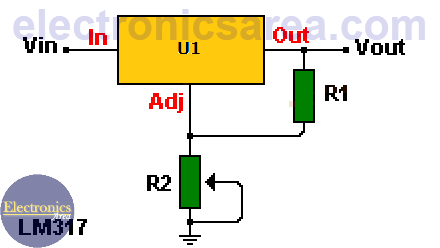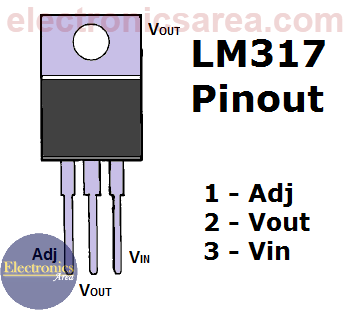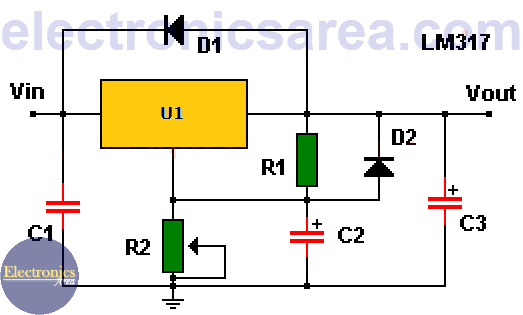# LM317 variable voltage regulator circuit

## LM117 / LM217 / LM317 variable voltage regulator characteristics

The LM317 is a 3-terminal positive voltage regulator with a 1.25 to 37 volts output voltage range. The LM317 regulator terminals are: IN (input), OUT (output), ADJ (adjust).

To achieve this voltage variation, only 2 external resistors are needed (one of them is a variable resistor). Two of the main characteristics of the LM317 voltage regulator are a current limitation and thermal protection against overloads.

## How the LM317 Variable Voltage Regulator circuit works?

The voltage between the ADJ and OUT terminals is always 1.25 volts (voltage established internally by the regulator) and consequently the current flowing through the resistor R1 is: IR1 = V/R1 = 1.25/R1. (1)The current through resistor R1 is IR1  and it is the same current that circulates through resistor R2 (current IADJ  is neglected). Then the voltage on resistor R2 is: VR2 = IR1 x R2 (2)

If IR1 (1) is substituted into the formula (2), the following equation is obtained: VR2 = 1.25 x R2/R1.

Since the output voltage is: Vout = VR1 + VR2, then …

Vout = 1.25 V + (1.25 x R2/R1) V.  ….. simplifying (common factor)
Vout = 1.25 V (1 + R2/R1) V.

From the last formula it is clear that if you modify the R2 resistor value (variable resistor), the Vout voltage is modified.

• R1 = 240 ohms
• R2 = 5K or 3K (potentiometer)The IADJ current was neglected, since it has a maximum value of 100uA and it remains constant with load and input voltage variations.

To improve the regulation, resistor R1 must be placed as close as possible to the regulator, while the R2 resistor ground terminal must be connected  as close as possible to the ground connection of the load.

## LM317 voltage regulator circuit operation improvement

In order to optimize the  LM317 variable voltage regulator circuit operation, some additional elements can be incorporated into the design. See the diagram below.LM317 / LM117 / LM217 variable voltage regulator using protection diodes

• A 0.1uF capacitor (C1) is placed at the input terminal (IN), if the voltage regulator is away from the rectification components.
• A 1uF tantalum capacitor or a 25uF electrolytic capacitor (C3) is placed at the output terminal (OUT) to improve the transient’s response.
• A 10uF electrolytic capacitor (C2) is placed in parallel with resistor R2, to improve rejection of the ripple voltage.
• A 1N4001 diode (D1) is placed to protect the regulator against possible short circuits at the regulator input.
• A 1N4001 diode (D2) is placed to protect the regulator against possible short circuits in its output when capacitors are discharged.

## What is the difference among LM117, LM217, LM317 voltage regulators?

LM117 operating temperature range: -55 and 150 °C
LM127 operating temperature range: -25 and 150 °C
LM317 operating temperature range: 0 and 125 °C

Some regulators of this family include an additional suffix. (example: LM317K)

Suffix K – voltage regulator with TO-3 package. Dissipates 20 W maximum with a 1.5 A current
Suffix H – voltage regulator with TO-39 package. Dissipates 2 W maximum with a 0.5 A current
Suffix T – voltage regulator with TO-220 package. Dissipates 20 W maximum with a 1.5 A current
Suffix E – voltage regulator with LLC package. . Dissipates 2 W maximum with a 0.5 A current
Suffix S – voltage regulator with TO-263 package. Dissipates 4 W maximum with a 1.5 A current

• 3
•
•
•
•
•
•
•
•
3
Shares
•
3
Shares
• 3
•
•
•
•
•
•
•
Tagged with: